# Data Reduction Technique: Principal Component Analysis in Azure Machine Learning## Introduction

We will be discussing one of the most common Data Reduction Technique named Principal Component Analysis in Azure
Machine Learning in this article. After discussing the basic cleaning techniques, feature selection techniques in
previous articles, now we will be looking at a data reduction technique in this article.

Data Reduction mechanism can be used to reduce the representation of the large dimensional data. By using a data
reduction technique, you can reduce the dimensionality that will improve the manageability and visualability of
data. Further, you can achieve similar accuracies.

## Different Types of Data Reduction

There are different data reduction techniques as shown in the following screenshot.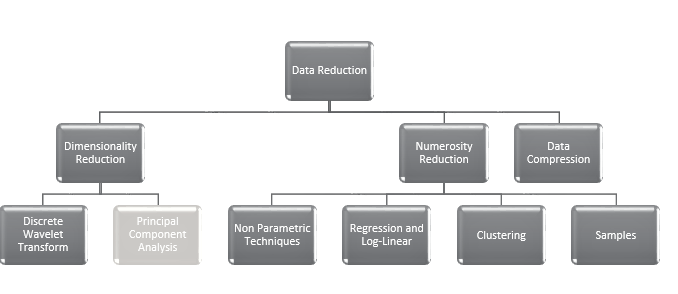You will see that there are many techniques for data reduction technique and Principal Component Analysis is a
Dimensionality reduction technique as highlighted in the above figure.

## What is Principal Component Analysis

Principal Component Analysis is also known as Karhunen-Loeve or K-L method, is used to reduce components to
handlable attributes from a large number of the dimensions. In other words, Principal Component Analysis combines
the important features of attributes and reduce the variables by introducing alternative variables. After the
Principal Component Analysis is done, multiple dimensions can be represented into a manageable number of variables.

## Principal Component Analysis in Azure Machine Learning

Let us see how we can use Principal Component Analysis in the Azure Machine Learning environment.

As we have been doing for the previous examples, let us drag the Adventureworks dataset to a newly created
experiment in the Azure Machine Learning. The following is the sample set of data.

You will see there are 28 columns and some of them are not going to make any contribution to the decision of buying
bikes. For example, Name, Address or email addresses are highly unlikely to contribute to the decision of bike
buying. Therefore, we need to eliminate these columns from the data flow. We will be using Select Columns in Dataset control. Please note that we discussed this control during the Data Cleaning article.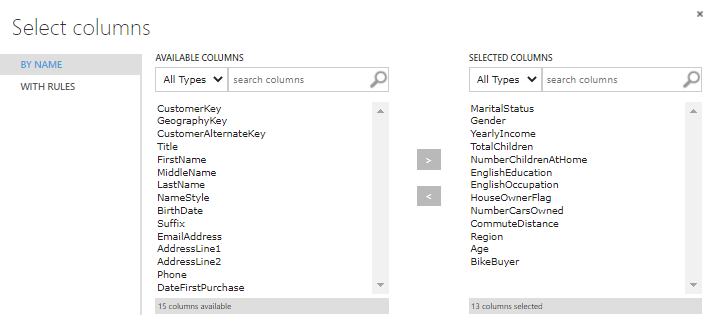As shown in the above screenshot, attributes such as Marital Status, Gender, Yearly Income, Total Children, Number
of Children at Home, Education, Occupation, House Owner Flag, Number of Cars owned, Commuted Distance, Region and
Age are selected independent variables along with the Dependent variable Bike Buyer.

Now let us include Principal Component Analysis in Azure Machine Learning in order to reduce the dimensionality.

Principal Component Analysis is located in the Data Transformation -> Scale and Reduce section
as shown in the below screenshot. Further, you can search for the Principal Component Analysis by using the search
option at the top of the controls.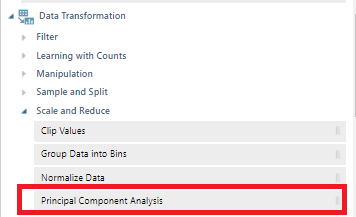Please note that we will be using Normalize Data control later in the article.

Let us configure the Principal Component Analysis in Azure Machine Learning as shown in the below screenshot.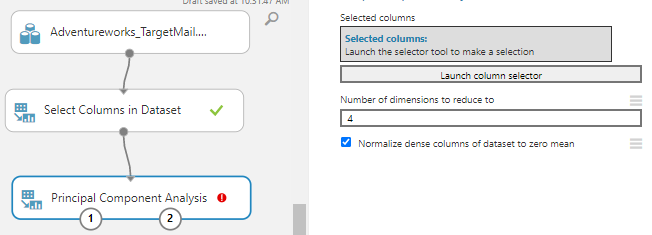We have configured the number of dimensions or components to 4. This means that all the selected variables will be
converted into four dimensions.

Next is to select attributes that should be converted to four components as we configured before.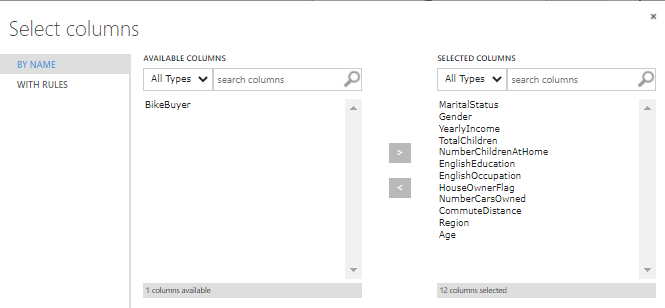In the above, we have selected all the independent variables as shown in the above screenshot. After executing the
experiment, you will see the following output in the first output of the Principal Component Analysis control.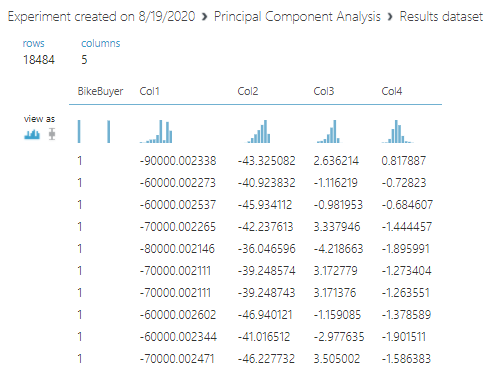Now, you will see that your all independent variables are replaced with four new independent variables. Let us
create the prediction model as we did in the previous article, Prediction in Azure Machine Learning.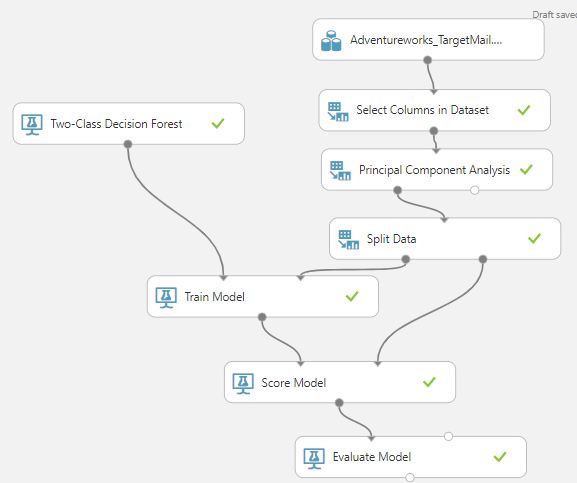Since we have discussed the creation of prediction models in previous articles, let us discuss the creation of a

First, let us split the data from Split Data control where 70% of data for training and the rest of
30% is to test the model. Then we will use the Train Model where the model is trained from
the Two-Class Decision Forrest algorithm. Then the score model control is included
to compare the test and finally, The Evaluate Model is added to evaluate the
created prediction model.

When you evaluate the created model, accuracy is 75.8% while the F1 score is 75.4%.

Let us compare the above results with the feature selection and without any feature selection which was discussed in
the previous article.

 With Feature Selection Without Feature Selection Principal Component Analysis Accuracy 0.725 0.801 0.758 Precision 0.727 0.796 0.757 Recall 0.712 0.802 0.751 F1 Score 0.720 0.799 0.754

You will observe that the principal component analysis in Azure Machine Learning is better than the Feature
selection but not accurate as all the features which are obvious.

## Normalization Data

You will observe that Col1 and Col2 have a different range of values from Col3 and Col below screenshot.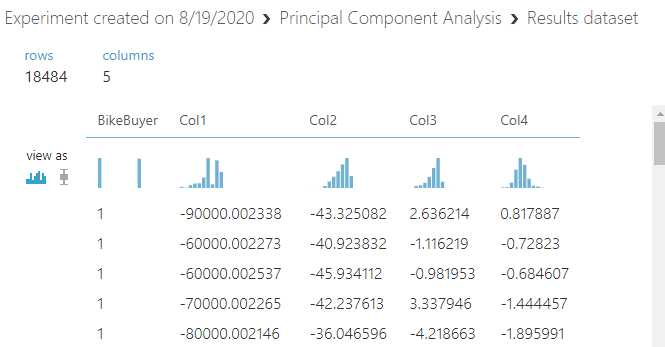This type of data set we can normalize the dataset using the Normalize Data
control as shown in the below screenshot.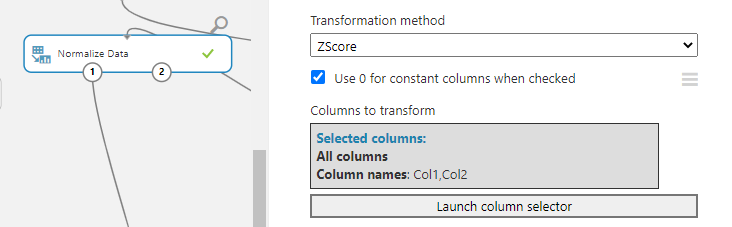In this, we have used the Zscore normalization method and we have selected Col1 and Col2 columns for transform. The
following is the screenshot for the output after the Transformation.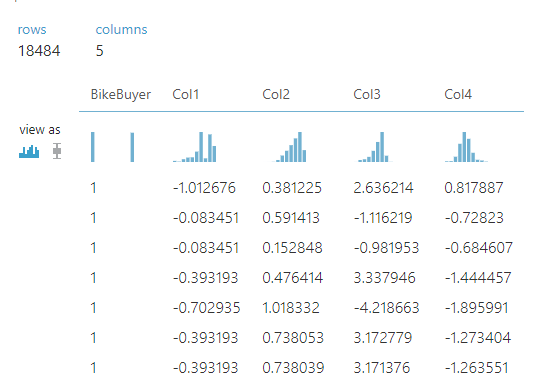Now you will see that all columns are normalized.

When the evaluation is done, like what we did last time, you will see that there is no difference between
normalization and without normalization.

 With Feature Selection Without Feature Selection Principal Component Analysis After Normalization Accuracy 0.725 0.801 0.758 0.758 Precision 0.727 0.796 0.757 0.757 Recall 0.712 0.802 0.751 0.751 F1 Score 0.720 0.799 0.754 0.754

This means there is no impact due to the Normalization. However, you could use the Normalization where necessary.

## Feature Selection and Principal Component Analysis

Now let us see how we can combine Feature selection that was
discussed in the last article with the Principal Component Analysis in Azure Machine Learning.

In the previous article, using different scoring techniques, we identified that we can choose the important
variables rather than selecting all the variables. For example, using Pearson correlation, we identified that Number
of Cars Owned, Total Children, Age, Number of Children at Home and Yearly Income are the most important variables
that will decide on the decision of bike buying. In that technique, we ignored all the other variables.

Keeping that in the count, let us select the only less important variables at the principal component analysis and
convert this to three components.

In that context, we have configured Principal Component Analysis in Azure Machine Learning as shown in the below screenshot.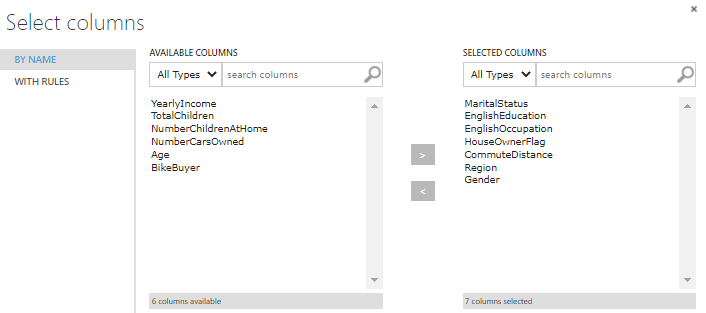The Following screenshot shows the output of the Principal Component analysis.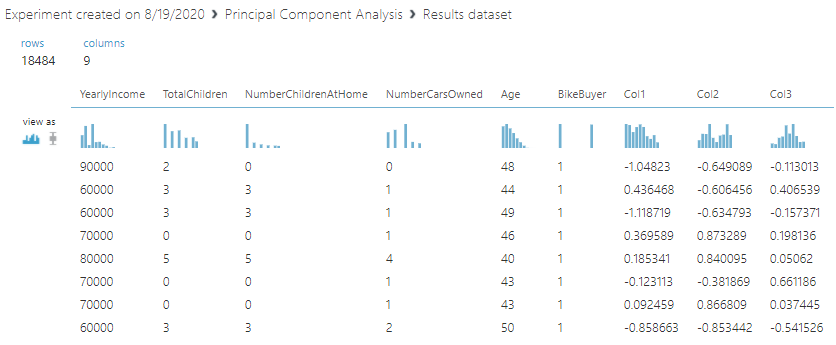Now you have converted the entire data set, the most important variables and three additional variables. Since all
values appear to be in the same range, there is no need for the normalization component as before.

 With Feature Selection Without Feature Selection Principal Component Analysis Feature Selection & Principal Component Analysis Accuracy 0.725 0.801 0.758 0.775 Precision 0.727 0.796 0.757 0.772 Recall 0.712 0.802 0.751 0.773 F1 Score 0.720 0.799 0.754 0.772

In the above table you will that, accuracy and other parameters are close to the Normal prediction to the feature
selection with Principal component Analysis in Azure Machine Learning. Further, this shows that the combination of
Feature Selection and Principal Component Analysis has better accuracy than they are considered separately.

## Conclusion

Principal Component Analysis in Azure Machine Learning is used to reduce the dimensionality of a dataset which is a
major data reduction technique. This technique can be implemented for a dataset with a large number of dimensions
such as surveys etc. Principal Components Analysis can be used along with the Feature Selection to achieve better
results.